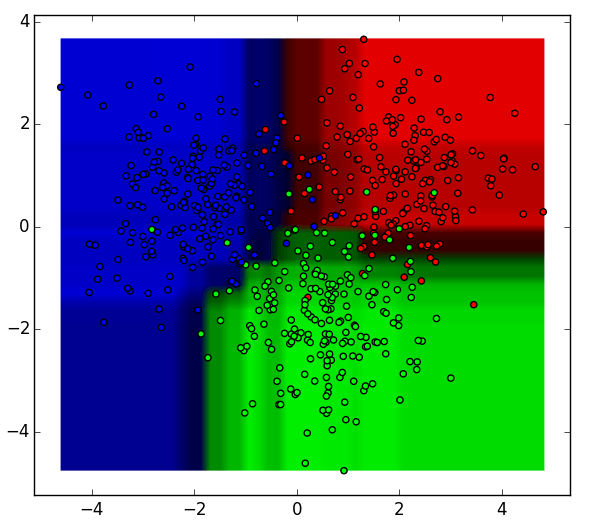# Random Forest Regression

Along with its implementation in Python

In this blog we’ll try to understand one of the most important algorithms in machine learning i.e. Random Forest Algorithm. We will try to look at the things that make Random Forest so special and will try to implement it on a real life dataset. The Code along with the dataset can be found here.

## Ensemble Learning

An Ensemble method is a technique that combines the predictions from multiple machine learning algorithms together to make more accurate predictions than any individual model. A model comprised of many models is called an Ensemble model.

## Types of Ensemble Learning:

1. Boosting.
2. Bootstrap Aggregation (Bagging).

### 1. Boosting

Boosting refers to a group of algorithms that utilize weighted averages to make weak learners into stronger learners. Boosting is all about “teamwork”. Each model that runs, dictates what features the next model will focus on.

In boosting as the name suggests, one is learning from other which in turn boosts the learning.

### 2. Bootstrap Aggregation (Bagging)

Bootstrap refers to random sampling with replacement. Bootstrap allows us to better understand the bias and the variance with the dataset. Bootstrap involves random sampling of small subset of data from the dataset. It is a general procedure that can be used to reduce the variance for those algorithm that have high variance, typically decision trees. Bagging makes each model run independently and then aggregates the outputs at the end without preference to any model.

### Problems with Decision Trees :(

Decision trees are sensitive to the specific data on which they are trained. If the training data is changed the resulting decision tree can be quite different and in turn the predictions can be quite different. Also Decision trees are computationally expensive to train, carry a big risk of overfitting, and tend to find local optima because they can’t go back after they have made a split. To address these weaknesses, we turn to Random Forest :) which illustrates the power of combining many decision trees into one model.

### Random Forest

Random forest is a Supervised Learning algorithm which uses ensemble learning method for classification and regression.

Random forest is a bagging technique and not a boosting technique. The trees in random forests are run in parallel. There is no interaction between these trees while building the trees.

It operates by constructing a multitude of decision trees at training time and outputting the class that is the mode of the classes (classification) or mean prediction (regression) of the individual trees.

A random forest is a meta-estimator (i.e. it combines the result of multiple predictions) which aggregates many decision trees, with some helpful modifications:

1. The number of features that can be split on at each node is limited to some percentage of the total (which is known as the hyperparameter). This ensures that the ensemble model does not rely too heavily on any individual feature, and makes fair use of all potentially predictive features.
2. Each tree draws a random sample from the original data set when generating its splits, adding a further element of randomness that prevents overfitting.

The above modifications help prevent the trees from being too highly correlated.

For Example, See these nine decision tree classifiers below :

These decision tree classifiers can be aggregated into a random forest ensemble which combines their input. Think of the horizontal and vertical axes of the above decision tree outputs as features x1 and x2. At certain values of each feature, the decision tree outputs a classification of “blue”, “green”, “red”, etc.

These above results are aggregated, through model votes or averaging, into a single ensemble model that ends up outperforming any individual decision tree’s output.

The aggregated result for the nine decision tree classifiers is shown below :Random Forest ensemble for the above Decision Tree classifiers

### Feature and Advantages of Random Forest :

1. It is one of the most accurate learning algorithms available. For many data sets, it produces a highly accurate classifier.
2. It runs efficiently on large databases.
3. It can handle thousands of input variables without variable deletion.
4. It gives estimates of what variables that are important in the classification.
5. It generates an internal unbiased estimate of the generalization error as the forest building progresses.
6. It has an effective method for estimating missing data and maintains accuracy when a large proportion of the data are missing.

### Disadvantages of Random Forest :

1. Random forests have been observed to overfit for some datasets with noisy classification/regression tasks.
2. For data including categorical variables with different number of levels, random forests are biased in favor of those attributes with more levels. Therefore, the variable importance scores from random forest are not reliable for this type of data.

## Implementation of Random Forest Regression on real life dataset:

### 2. Splitting our dataset into training set and test set

(It’s just for illustration purpose. There is no need to split the dataset over here since we only have 10 values in it)

### 3. Creating a Random Forest Regression model and fitting it to the training data

For this model I’ve chosen 10 trees (n_estimator=10)

### Conclusion :

So here you go, we have learned about the various kinds of ensemble learning algorithms and how these algorithms help in making Random Forest work. We have got an intuitive understanding of the features and advantages of using Random Forest over other Machine Learning algorithms. Apart from it we also saw some of its disadvantages along with its implementation on a actual dataset.